Next:2. ListsUp:1. IntroductionPrevious:1.3 To Probe Further

# 1.4 Problems

1.
Assuming that n is a power of 2, express the output of the following program in terms of n.
         int mystery (int n)
{ int x=2, count=0;
while (x < n) { x *=2; count++}
return count;
}
2.
Show that the following statements are true:
(a)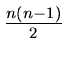is O(n2)
(b)
max(n3, 10n2) is O(n3)
(c)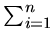ik is O(nk + 1) and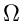(nk + 1), for integer k
(d)
If p(x) is any kth degree polynomial with a positive leading coefficient, then p(n) is O(nk) and(nk).
3.
Which function grows faster?
(a)
nlog n(logn)n
(b)
lognk(logn)k
(c)
nlogloglog n; (log n)!
(d)
nn; n!.
4.
If f1(n) is O(g1(n)) and f2(n) is O(g2(n)) where f1and f2 are positive functions of n, show that the function f1(n) + f2(n) is O(max(g1(n), g2(n))).
5.
If f1(n) is O(g1(n)) and f2(n) is O(g2(n)) where f1and f2 are positive functions of n, state whether each statement below is true or false. If the statement is true(false), give a proof(counter-example).
(a)
The function | f1(n) - f2(n)| is O(min(g1(n), g2(n))).
(b)
The function | f1(n) - f2(n)| is O(max(g1(n), g2(n))).
6.
Prove or disprove: If f (n) is a positive function of n, then f (n) is O(f (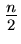)).
7.
The running times of an algorithm A and a competing algorithm A' are described by the recurrences
T(n) = 3T(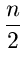) + n;        T'(n) = aT'(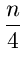) + n

respectively. Assuming T(1) = T'(1) = 1, and n = 4k for some positive integer k, determine the values of a for which A' is asymptotically faster than A.
8.
Solve the following recurrences, where T(1) = 1 and T(n) for n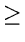2 satisfies:
(a)
T(n) = 3T() + n
(b)
T(n) = 3T() + n2
(c)
T(n) = 3T() + n3
(d)
T(n) = 4T(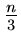) + n
(e)
T(n) = 4T() + n2
(f)
T(n) = 4T() + n3
(g)
T(n) = T() + 1
(h)
T(n) = 2T() + log n
(i)
T(n) = 2T() + n2
(j)
T(n) = 2T(n - 1) + 1
(k)
T(n) = 2T(n - 1) + n
9.
Show that the function T(n) defined by T(1) = 1 and
T(n) = T(n - 1) +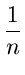for n2 has the complexity O(log n).
10.
Prove or disprove: f(3**n) is O(f(2**n))
11.
Solve the following recurrence relation:
T(ncn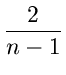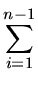T(i)

where c is a constant, n2, and T(2) is known to be a constant c1.Next:2. ListsUp:1. IntroductionPrevious:1.3 To Probe Further
eEL,CSA_Dept,IISc,Bangalore